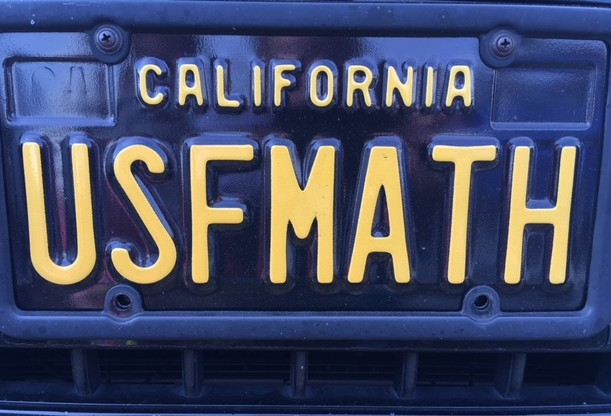Mathematics

Article

2012

Abstract

In this paper we derive evolution equations for the two-dimensional active scalar problem when the solution is supported on one-dimensional curves. These equations are a generalization of the Birkhoff–Rott equation when vorticity is the active scalar. The formulation is Lagrangian and it is valid for nonlocal kernels K that may include both a gradient and an incompressible term. We develop a numerical method for implementing the model which achieves second order convergence in space and fourth order in time. We verify the model by simulating classic active scalar problems such as the vortex sheet problem (in the case of inviscid, incompressible flow) and the collapse of delta ring solutions (in the case of pure aggregation), finding excellent agreement. We then study two examples with kernels of mixed type, i.e., kernels that contain both incompressible and gradient flows. The first example is a vortex density model which arises in superfluids. We analyze the effect of the added gradient component on the Kelvin–Helmholtz instability. In the second example, we examine a nonlocal biological swarming model and study the dynamics of density rings which exhibit complicated milling behavior.

Copyright 2012 Society for Industrial and Applied Mathematics

Article available at: http://dx.doi.org/10.1137/110819883

DOI

10.1137/110819883

COinS